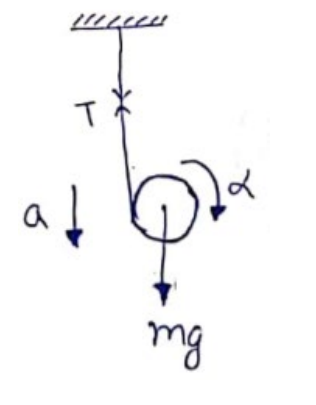# Solve the following :Question:

A string is wrapped over the edge of a uniform disc and the free end is fixed with the ceiling. The disc moves down, unwinding the string. Find the downward acceleration of the disc.

Solution:$\mathrm{mg}-\mathrm{T}=\mathrm{ma}-(\mathrm{i})$

$\mathrm{T}=\mid \alpha$

$T \cdot R=\frac{m R^{2}}{2}\left(\frac{a}{R}\right)$

From (i) and (ii)

$a=\frac{2 g}{3}$